# Chi-Square Distribution

(redirected from Chi-squared distribution)
Also found in: Dictionary, Medical, Wikipedia.
Related to Chi-squared distribution: T distribution, Beta distribution

## chi-square distribution

[′kī ¦skwer dis·trə′byü·shən]
(statistics)
The distribution of the sum of the squares of a set of variables, each of which has a normal distribution and is expressed in standardized units.
McGraw-Hill Dictionary of Scientific & Technical Terms, 6E, Copyright © 2003 by The McGraw-Hill Companies, Inc.
The following article is from The Great Soviet Encyclopedia (1979). It might be outdated or ideologically biased.

## Chi-Square Distribution

The probability distribution of the sum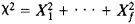of the squares of the normally distributed random variables X1, . . ., Xf, with zero mathematical expectation and unit variance is known as a chi-square distribution with f degrees of freedom. The distribution function for a chi-square random variable is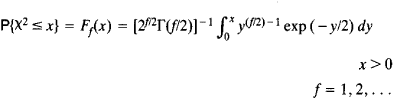The first three moments (the mathematical expectation, the variance, and the third central moment) of χ2 are f, 2f, and 8f, respectively. The sum of two independent random variables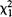and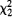with f1 and f2 degrees of freedom has a chi-square distribution with f1 + f2 degrees of freedom.

Examples of chi-square distributions are the distributions of the squares of random variables that obey the Rayleigh and Maxwellian distributions. The Poisson distribution can be expressed in terms of a chi-square distribution with an even number of degrees of freedom: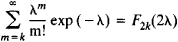If the number f of terms of the sum χ2 increases without bound, then, according to the central limit theorem, the distribution function of the standardized ratioconverges to the standard normal distribution: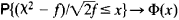where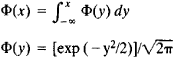A consequence of this fact is another limit relation, which is convenient for calculating Ff(x) when f has large values: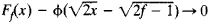In mathematical statistics, the chi-square distribution is used to construct interval estimates and statistical tests. Let Yi, . . ., Yn be random variables representing independent measurements of an unknown constant a. Suppose the measurement errors Yia are independent and are distributed identically normally. We have

E(Yia) = 0 E(Yia)2 = σ2

The statistical estimate of the unknown variance σ2 is then expressed by the equation

s2 = S2/(n – 1)

where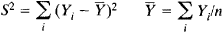The ratio S22 obeys a chi-square distribution with f = n – 1 degrees of freedom. Let x1 and x2 be positive numbers that are solutions of the equations Ff(x1) = α/2 and Ff(x2) = 1 – α/2, where α is a specified number in the interval (0,1/2). In this case

P{x1 < S22 < x2} = P{S2/x2 < σ2 < S2/x1} = 1 – α

The interval (S2/x1, S2/x2 is called the confidence interval for σ2 with confidence coefficient 1 – α.

This method of constructing an interval estimate for σ2 is often used to test the hypothesis that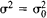, where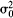is a given number. Thus, ifbelongs to the confidence interval indicated, then one concludes that the measurements do not contradict the hypothesis. If, however,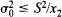or, then it must be assumed that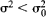or, respectively. This test corresponds to a significance level equal to α.

### REFERENCE

Cramer, H. Matematicheskie metody statistiki, 2nd ed. Moscow, 1975. (Translated from English.)

L. N. BOLSHEV

References in periodicals archive ?
The fault detection rate of using kernel density estimation is slightly superior to the chi-squared distribution.
It is well-known that the diversity order (corresponding to a slope of an error performance curve) is n when the SNR is distributed as a chi-squared distribution with 2n degrees of freedom .
Equation (33) implies that, for large K, the long-term throughput can be obtained by a single user scheduling problem in which each MS has a diversity order of ([N.sub.T] - N - L + 1), as dictated by Theorem 5 and therefore, it follows the chi-squared distribution with 2([N.sub.T] - N - L + 1) degrees of freedom.
The best-fit model for step length distribution is the chi-squared distribution, which is consistent with Brownian motion or a correlated random walk.
For time-based step lengths, we considered several models: uniform distribution (an uninformative null model); Levy as a Pareto distribution; and Brownian and correlated random as exponential, Rayleigh, and chi-squared distributions. After MLE, models were compared using their respective Akaike Information Criteria (AIC), given by AIC = 2k - 2ln (L), where L is the maximum value of the likelihood function for the model and k is the number of independently adjusted parameters within the model (Akaike, 1974; Burnham and Anderson, 2002; Evangelista et al., 2014).
Similar results were reported by PINTO (2009), who observed that the power values of the LRT (for a specific covariance) with exact distribution and of the LRT with approximate chi-squared distribution were similar when the sample size was increased to 50 and 100 observations.
This result shows that [MATHEMATICAL EXPRESSION NOT REPRODUCIBLE IN ASCII] is not asymptotically normally distributed but instead [MATHEMATICAL EXPRESSION NOT REPRODUCIBLE IN ASCII] has a weighted chi-squared distribution. Appendix B provides detailed derivations.
The chi-squared distribution. John Wiley and Sons, New York, New York, USA.
where [[chi square].sub.1]([delta]) is the upper [delta]-quantile of the chi-squared distribution with degrees of freedom equal to 1.
If the modified model is correct, then D (the deviance) has an approximate chi-squared distribution with K - 2 degrees of freedom.
However, by performing a series of [10.sup.5] randomizations, generating sets of inter-species distances from a uniform distribution, I have found that use of the critical values of the chi-squared distribution results in an inflated Type II error rate, particularly for low numbers of species (Table 1).

Site: Follow: Share:
Open / Close